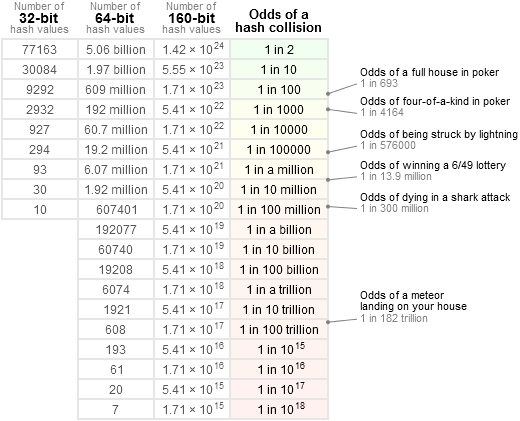# Mathematics - Permutation (Ordered Combination)

A permutation is a selection of elements from a set where the order of selection matters.

Order matters means that the selections AB and BA are considered as two differents selections.

If the order does not matter such as in a lottery, the selections AB and BA would be considered as a the same selection and is known as combination

## Example

• with the set {1,2,3}
• with a selection without_repetition (where the elements are deleted from the set when selected)
• the possible permutations are:
• 1 2 3
• 1 3 2
• 2 1 3
• 2 3 1
• 3 1 2
• 3 2 1

If the order would have not matter, all this selections would have been seen as a single combination 1 2 3 (because the order in the selections does not matter)

## Type

There are basically two types of permutation:

• With Repetition is Allowed (ie AA): lock, pin, surrogate identifier
• Without Repetition (ie the pair AA is not allowed): the first three people in a running race (a participant can't be first and second), all queue problems.

### With repetition

• When a set has n different elements,
• For each choice, there is n choice,

For a set of length n and a selection of length r (known as k for combination), the number of permutation $_nP_r \text{ (n permute r)}$ is: $$_nP_r = n_1 \times n_2 \dots \times n_r = n^r$$

### Without repetition

• When a set has n different elements,
• For the choice i, there is n-i+1 choice,

For a set of length n and a selection of length k, the number of permutation is: $$_nP_k = \frac{n!}{(n-k)!}$$

The division (n-k)! is a trick to be able to generalize the calculation with factorial

Example: if for a set of 10 element, with a selection of 3 elements, we can choose for:

• the first choice: 10 elements (we delete the selected element of the set)
• the second choice: 9 elements (we delete the selected element of the set)
• the third choice: 8

In total, we have: $$10 * 9 * 8 \text{ permutations}$$

To generalize with the factorial, we add the rest of the factorial serie in the dividend and divisor:

$$10 \times 9 \times 8 \times \frac{ 7 \times 5 \times 4 \times 3 \times 2 \times 1}{7 \times 5 \times 4 \times 3 \times 2 \times 1} = \frac{10!}{(10-3)!} = \frac{n!}{(n-k)!}$$

## Documentation / Reference

Recommended PagesCollisions of Hash or Identifier Generation

A collision happens when a function produces the same result while it should be unique. In a hash function, it happens when two different inputs produces the same hash In a identifier generator, it...Mathematics - Combination (Binomial coefficient|n choose k)

A combination is a selection of elements from a set where the order of selection does not matter. Order doesn't matter means that the selections AB and BA are considered a single combination (a single...Mathematics - Exponentiation (square, cube) - Power

Exponentiation is a binary operation involving two numbers: the base (b) the exponent (n) (or index or power). In text notation or computer language, generally the exponentiation operator...